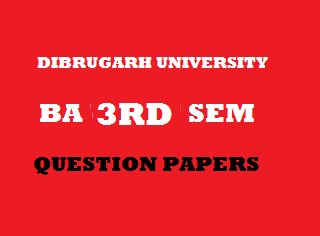## Friday, January 04, 20192013
(November)
MATHEMATICS
(Major)
Course: 302
(Coordinate Geometry and Algebra – I)
Full Marks: 80
Pass Marks: 32
Time: 3 hours
The figures in the margin indicate full marks for the questions
GROUP – A
(Coordinate Geometry)
SECTION – I
(2-Dimension)
(Marks: 27)

1. (a) What will be the equation of the linewhen the origin is transferred to the point1
(b) Transform the equationreferred to new axes throughrotated through an angle4
Or
Find the transformed equation of the curvewhen the two perpendicular linesandare taken as coordinate axes.
2. (a) Interpret the situation for the straight lines given bywhen1
(b) Prove that the lines represented byhave the same pair of bisectors for all values of. 2
(c) Show that the general equation of 2nd degreerepresents a pair of parallel straight lines if4
Or
Find the condition that one of the lines given bymay be perpendicular to one of the lines given by.
(d) Ifrepresents a pair of lines, then prove that the square of the distance of their point of intersection from the origin is5
Or
Ifrepresents a pair of lines, then prove that the product of the perpendiculars from the origin on these lines is3. (a) State True or False: A parabola has its centre at infinity. 1
(b) From the equation of the diameter of the conicconjugate to the diameter3
(c) Define a conic section. Reduce the equationto the standard form.         1+5=6
Or
Find the equation of the polar of a given pointwith respect to the conic 6SECTION – II
(3-Dimension)

4. (a) State the intercepts made on the axes by the plane1
(b) Find the equation of the plane through the pointsandand parallel to the y-axis.    3
(c) Write the equation of the line through the pointparallel to the z-plane. 1
(d) Put the equationsof a line in the symmetrical form. 5
Or
Find the equation of the plane through the lineparallel to the line5. (a) Fill up the blank: 1
If the shortest distance between two lines is zero, then the lines are ____.
(b) Find the shortest distance between the y-axis and the line 2(c) Find the shortest distance between the linesandAnd the equation of the line that represents shortest distance. 5
Or
Find the length and equations of the line of the shortest distance between the linesandGROUP – B
(Algebra – I)
(Marks: 35)

6. (a) State True or False: 1
“A mapis invertible iff it is one-one into.”
(b) Give an example to show that a coset may not be a subgroup of a group. 1
(c) Ifbe a group of prime order, then show thathas no proper subgroup. 2
(d) Answer any two questions: 3x2=6
1. Show that an infinite cyclic group has precisely two generators.
2. Let H, K be subgroups of G. Show that HK is a subgroup of G if and only if HK = KH.
3. Let G be a group. Show thatwhere n is an integer and7. Answer any two questions: 5x2=10
1. Prove that the set of matriceswhereis a real number, forms a group under matrix multiplication.

1. Show that if G is a group of order 10, then it must have a subgroup of order 5.
2. State and prove Lagrange’s theorem.
8. (a) Define normal subgroup. 1
(b) If G is a finite group and N is a normal subgroup of G, then prove that 2(c) Ifbe a homomorphism, then prove that Keris a normal subgroup of3
9. Answer any one question: 4
(a) Ifis a homomorphism, then prove that -
1.2.;an integer whereandare identify elements ofandrespectively.
(b) If H and K are two subgroups which are not normal subgroups, then HK is a normal subgroup. Justify with an example.
10. Answer any one question: 5
1. Show that a subgroup H of a group G is normal in G if and only if1. Show that every group is isomorphic to a permutation group.

***